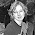jueves, 13 de septiembre de 2012

Extra points 1

The last week I choosed explain the following expressions:

c = me mod n

and

m = cd mod n

For this example I used  m = 123(message), n = 3233, d = 2753.

c = 12317mod 3233 = 855
m = 8552753mod 3233 = 123

The property that make RSA invertible is:

med-1≡1 mod n

To get the message after encription we have de following relations(ku -public key, kr-private key)

Dkr(Eku(m)) = (mmod n)d mod n ⇔ m ≡ med mod n
⇔ 1 ≡ med-1 mod n

12317 mod 3233 = 855 ⇔ 123 =12317*2753 mod 3233 ⇔ 1 = 12317*2753-1 mod 3233

We need to be sure that  the values of e, d, n satisfy:

∀ m ∈ Z : med-1 ≡ 1 mod n

Now is possible note that the first two expressions work in noth directions.
When we have decryption first and then encryption, the expression is equal to:

Eku(Dkr(c)) = c de mod n ≡ c mod n

8552753*17 mod 3233 ≡ 855 mod 3233 = 855

This is the correctness in the two directions.

References

Applied Cryptography

2 comentarios:

1.But why does m^(ed) give m in mod n given that ed = 1 in mod phi(n)? 1 extra point awarded.

2.Thanks for the above tutorial. It helps me a lot in my studies.Please help me in other expressions also. I discussed this blog with my friends also.They also want to know more information. So please share.
PDF signature